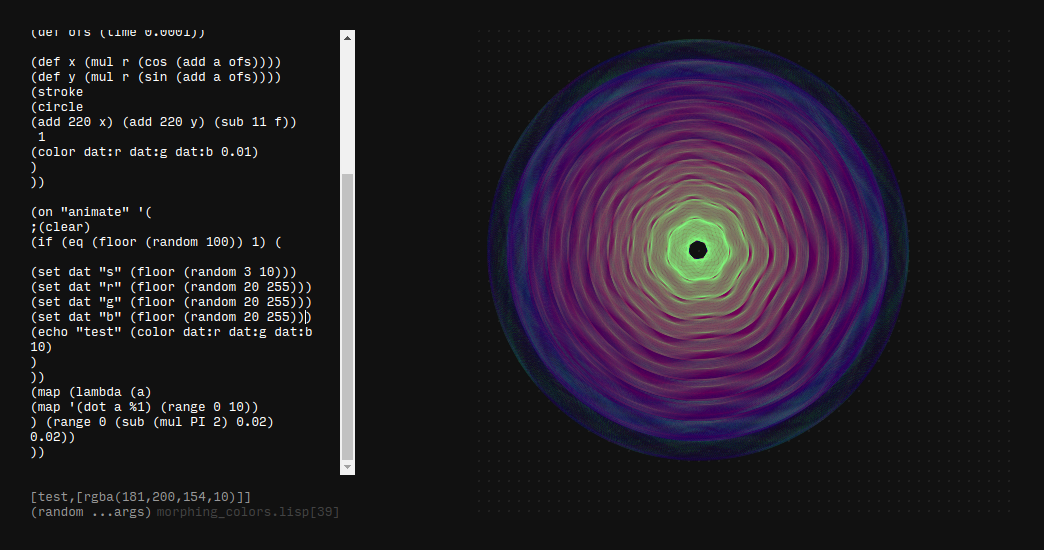## Ronin experiment 10 - morphing colors

I created some rotating flower shapes to create layers of colors that slowly mix into a colorfull circle similar to batik t-shirts from the 60'sthis is the ronin script i used to create the colors

``````(def dat {:s 5 :r 55 :g 11 :b 255 })

(defn color (r g b a) (
(concat "rgba(" r "," g "," b "," a ")")
))

(defn dot (a f) (
(def r
(add (mul 20 f) (mul f (sin (mul a dat:s ))))
)
(def ofs (time 0.0001))

(def x (mul r (cos (add a ofs))))
(def y (mul r (sin (add a ofs))))
(stroke
(circle
1
(color dat:r dat:g dat:b 0.02)
)
))

(on "animate" '(
;(clear)
(if (eq (floor (random 20)) 1) (

(set dat "s" (floor (random 3 10)))
(set dat "r" (floor (random 20 255)))
(set dat "g" (floor (random 20 255)))
(set dat "b" 0)
(echo "test" (color dat:r dat:g dat:b 10)
)
))
(map (lambda (a)
(map '(dot a %1) (range 0 10))
) (range 0 (sub (mul PI 2) 0.02) 0.02))
))
``````